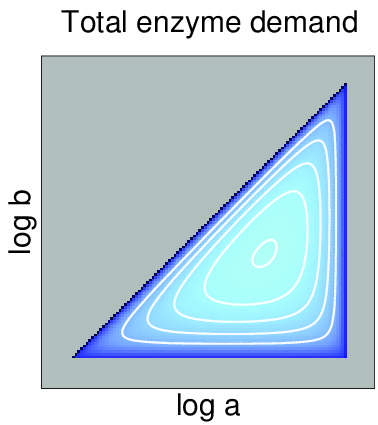# Enzyme cost minimization#### Enzyme cost minimization

Enzyme cost plays a major role in the choice of metabolic routes, both in evolution and bioengineering. If the desired fluxes are known, minimal requires enzyme levels, as well as corresponding metabolite levels, can be predicted based on known rate laws and on a principle of minimal enzyme cost.
The numerical calculations are greatly facilitated by formulating this as a convex optimality problem. The set of feasible metabolite profiles forms a polytope in log-concentration space, whose points represent all possible steady states of a kinetic model. Enzyme cost is a convex function on this polytope. The resulting method - finding optimal enzyme profiles and corresponding metabolite profiles that realize a desired flux at a minimal cost - is called enzyme cost minimization (ECM).

Aside from being a practical simulation method, ECM also yields some theoretical insights. The optimal enzyme profiles depend on kinetic rates laws and are therefore shaped by factors such as catalytic constants, thermodynamic driving forces, enzyme saturation, and allosteric regulation. The enzymatic cost can be split into multiplicative contributions from enzyme capacity, energetics, and kinetics. By neglecting some of these factors, we construct simplified enzyme cost functions, applicable even if few or no parameter values are known. The energetic cost contribution is directly linked to thermodynamic constraints: if a reaction is thermodynamically unfeasible, we can simply see this as a case of infinitely high enzyme cost.
The enzyme costs computed by ECM lead to general flux cost functions, which can be used to bridge the gap between constraint-based and kinetic metabolic models. Finally, the optimality condition of ECM can be interpreted as a balance relation between the costs of adjacent enzymes. This links flux analysis to metabolic control analysis.

On this website, you can find software for enzyme cost minimization (in matlab and python), as well as data files for an illustrative example, a kinetic model of central carbon metabolism in E. coli.

### References

If you use enzyme cost minimization in your work, please cite our articles

Noor E., Flamholz A., Bar-Even A., Davidi D., Milo R., Liebermeister W. (2016), The protein cost of metabolic fluxes: prediction from enzymatic rate laws and cost minimization, PLoS Comp. Biol. 12 (10): e1005167

Metabolic enzyme cost explains variable trade-offs between microbial growth rate and yield, Wortel M.T., Noor E., Ferris M., Bruggeman F.J., Liebermeister W. (2018), PLoS Computational Biology 14(2): e1006010